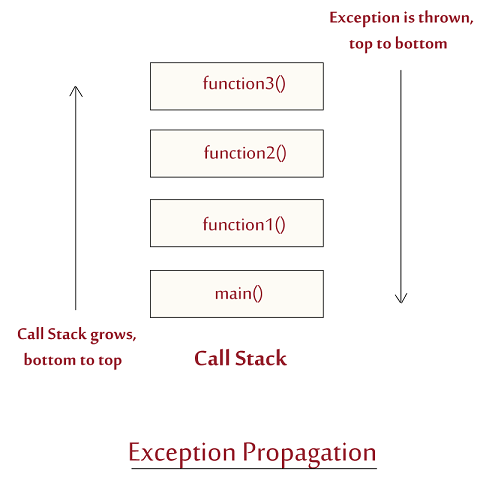< Prev
Next >

# C++ Exception Propagation

Do you know what happens when an exception occurs in our program and how it travels through our program after it is originated? Let's continue reading to know about how exactly an exception propagates through our code after it is raised.

## What happens when an exception is thrown by the program?

Considering a scenario, in which your program starts its execution at the main() function, main() calls function1(), function1() calls function2(), function2() calls function3(), now the call stack should look like the figure below-:The currently executing function is at the top of the call stack, which in this example is function3(). Let's say while executing function3(), an exception is raised/thrown. Now this exception is thrown down to the next function in the call stack, i.e. function2(), which in turn throws the exception down to next function in the call stack, i.e. function1(). The process continues until the exception is thrown to the function at the bottom of the call stack, i.e. main() function.

Now, at this time call stack explodes which leads to the abrupt termination of the program.

## Exception propagation example

Exp.cpp
``````#include<iostream>

using namespace std;

class Excp
{
public:
void function1();
void function2();
void function3();
};

void Excp :: function1()
{
function2();
};

void Excp :: function2()
{
function3();
}

void Excp :: function3()
{
int a = 20;
int b = 20;
int c = x/(x-y);   //This will throw an exception because we are dividing a number with zero i.e. x-y is 0
cout<<"Hello";     //This statement will not be executed.
}

int main()
{
Excp ob;
ob.function1();
}``````

In the program above, the main() functions calls the function1() function, which calls function2() function, which in turn calls the function3() function. Within the function3() function, compiler finds a division-by-zero problem which is raised when a integer number is divided by zero, hence an exception of type int is thrown.

The function3() throws this uncaught exception down to the next function in the call stack, i.e. function2(), which throws the uncaught exception down the next function in the call stack, i.e. function1() and the process continues until this uncaught exception reaches the function at the bottom of the call stack, i.e. main() function.

At this time, the exception remains uncaught and the program ends abruptly because we have not caught the exception, hence,std::terminate() will be called, which will terminate the execution of the program with a message - Exp.exe has stopped working.

Hence, it is important to handle the exception in a program, so that an exception doesn't alter the normal flow of the program and ends it unexpectedly.

The process of handling an exception in a program is called Exception Handling. We will learn about strategy of Exception Handling using try, catch blocks and throw keyword in the next article.

< Prev
Next >
< What is Exception?
Try-Catch block >